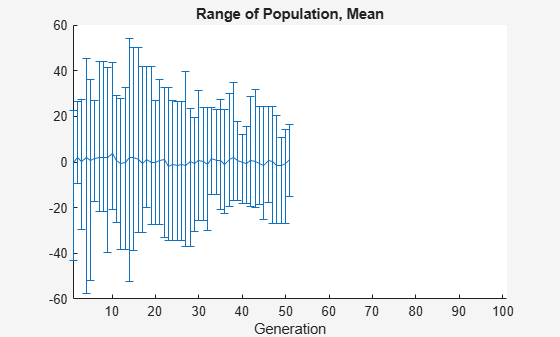# Global vs. Local Optimization Using `ga`

### Searching for a Global Minimum

Sometimes the goal of an optimization is to find the global minimum or maximum of a function—a point where the function value is smaller or larger at any other point in the search space. However, optimization algorithms sometimes return a local minimum—a point where the function value is smaller than at nearby points, but possibly greater than at a distant point in the search space. The genetic algorithm can sometimes overcome this deficiency with the right settings.

As an example, consider the following function.

`$f\left(x\right)=\left\{\begin{array}{ll}-\mathrm{exp}\left(-{\left(\frac{x}{100}\right)}^{2}\right)& for\phantom{\rule{0.5em}{0ex}}x\le 100,\\ -\mathrm{exp}\left(-1\right)+\left(x-100\right)\left(x-102\right)& for\phantom{\rule{0.5em}{0ex}}x>100.\end{array}$`

Plot the function.

```t = -10:.1:103; for ii = 1:length(t) y(ii) = two_min(t(ii)); end plot(t,y)```The function has two local minima, one at , where the function value is –1, and the other at , where the function value is . Since the latter value is smaller, the global minimum occurs at .

### Run `ga` Using Default Parameters

The code for the `two_min` helper function is at the end of this example. Run `ga` with default parameters to minimize the `two_min` function. Use the `gaplot1drange` helper function (included at the end of this example) to plot the range of the `ga` population at each iteration.

```rng default % For reproducibility options = optimoptions('ga','PlotFcn',@gaplot1drange); [x,fval] = ga(@two_min,1,[],[],[],[],[],[],[],options)```
```Optimization terminated: average change in the fitness value less than options.FunctionTolerance. ``````x = -0.0688 ```
```fval = -1.0000 ```

The genetic algorithm returns a point very close to the local minimum at . Note that all individuals lie between –60 and 60. The population never explores points near the global minimum at .

### Increase Initial Range

One way to make the genetic algorithm explore a wider range of points—that is, to increase the diversity of the populations—is to increase the initial range. The initial range does not have to include the point x = 101, but it must be large enough so that the algorithm generates individuals near x = 101. Set the `InitialPopulationRange` option to `[-10;90]` and rerun the solver.

```options.InitialPopulationRange = [-10;90]; [x,fval] = ga(@two_min,1,[],[],[],[],[],[],[],options)```
```Optimization terminated: maximum number of generations exceeded. ``````x = 100.9783 ```
```fval = -1.3674 ```

This time, the custom plot shows a much wider range of individuals. There are individuals near 101 from early on, and the population mean begins to converge to 101.

### Helper Functions

This code creates the `two_min` helper function.

```function y = two_min(x) if x <= 100 y = -exp(-(x/100)^2); else y = -exp(-1) + (x-100)*(x-102); end end```

This code creates the `gaplot1drange` helper function.

```function state = gaplot1drange(options,state,flag) %gaplot1drange Plots the mean and the range of the population. % STATE = gaplot1drange(OPTIONS,STATE,FLAG) plots the mean and the range % (highest and the lowest) of individuals (1-D only). % % Example: % Create options that use gaplot1drange % as the plot function % options = optimoptions('ga','PlotFcn',@gaplot1drange); % Copyright 2012-2014 The MathWorks, Inc. if isinf(options.MaxGenerations) || size(state.Population,2) > 1 title('Plot Not Available','interp','none'); return; end generation = state.Generation; score = state.Population; smean = mean(score); Y = smean; L = smean - min(score); U = max(score) - smean; switch flag case 'init' set(gca,'xlim',[1,options.MaxGenerations+1]); plotRange = errorbar(generation,Y,L,U); set(plotRange,'Tag','gaplot1drange'); title('Range of Population, Mean','interp','none') xlabel('Generation','interp','none') case 'iter' plotRange = findobj(get(gca,'Children'),'Tag','gaplot1drange'); newX = [get(plotRange,'Xdata') generation]; newY = [get(plotRange,'Ydata') Y]; newL = [get(plotRange,'Ldata') L]; newU = [get(plotRange,'Udata') U]; set(plotRange,'Xdata',newX,'Ydata',newY,'Ldata',newL,'Udata',newU); end end```